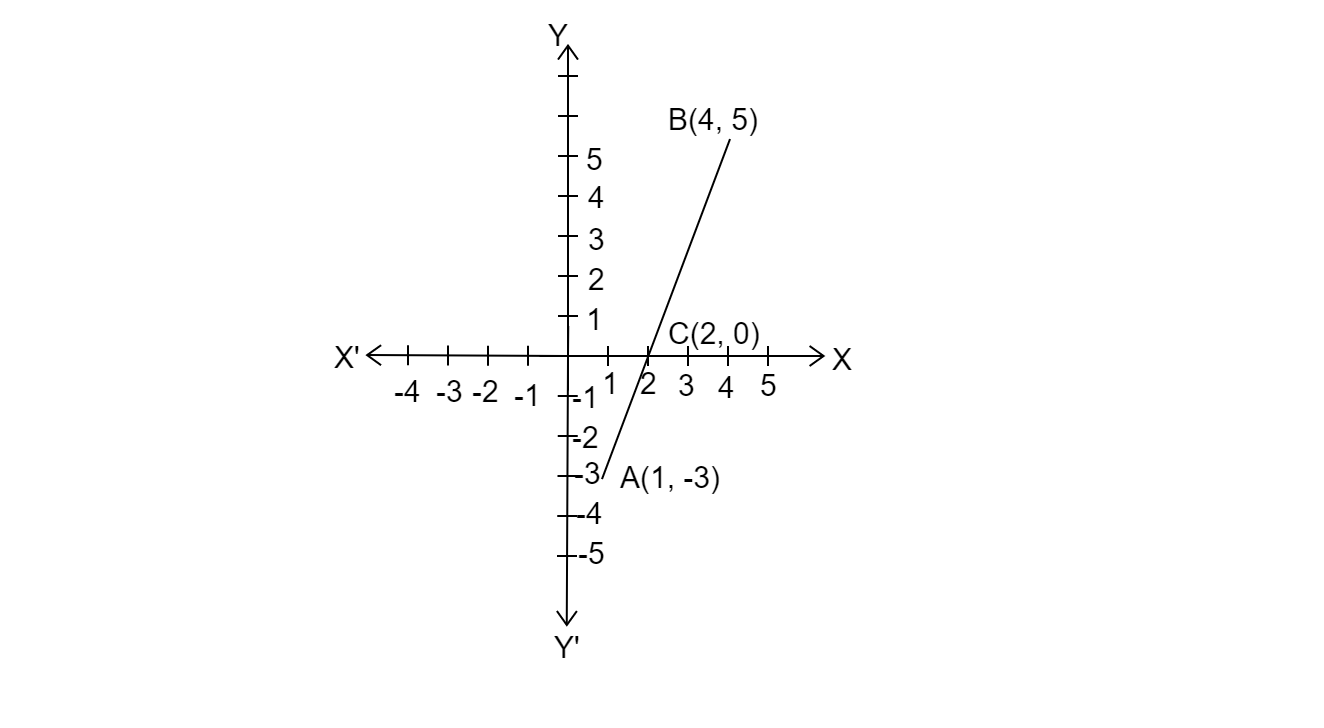# Find the ratio in which the segment joining the points $( 1,\ -3)$ and $( 4,\ 5)$ is divided by $x-$axis? Also find the coordinates of this point on $x-$axis.

Given: The segment joining the points $( 1,\ -3)$ and $( 4,\ 5)$ is divided by $x-$axis.

To do: To find the ratio of division and also to find the coordinates of this point on x-axis.

Solution:Let $C( x,\ 0)$ divides the line segment joining the points $A( 1,\ 3)$ md B(4,5) in k:1 ratio.

Using section formula, we have,

$C(x, y)=(\frac{nx_{1}+mx_{2}}{m+n}, \frac{ny_{1}+my_{2}}{m+n})$

$(x, 0)=(\frac{4k+1}{k+1}, \frac{5k+1(-3)}{k+1})$

$(x, 0)=(\frac{4k+1}{k+1}, \frac{5k-3)}{k+1})$

$\Rightarrow x=\frac{4k+1}{k+1} .............( 1)$

And $\frac{5k-3}{k+1}=0$

$\Rightarrow5k-3=0$

$\Rightarrow5k -3=0$

$\Rightarrow k=\frac{3}{5}$

On subtituting $k=\frac{3}{5}$ in $( 1)$,

$x=\frac{4(\frac{3}{5})+1}{(\frac{3}{5})+1}$

$x=\frac{\frac{17}{5}}{\frac{8}{5}}=\frac{17}{8}$

Hence the division ratio is $3:5$ and the co-ordinates of the point of division is $( x, 0)=( \frac{17}{8},\ 0)$

Updated on: 10-Oct-2022

49 Views